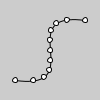# bezier_point()#

Evaluates the Bezier at point t for points a, b, c, d.

## Examples#```def setup():
py5.no_fill()
py5.bezier(85, 20, 10, 10, 90, 90, 15, 80)
py5.fill(255)
steps = 10
for i in range(0, steps+1):
t = i / steps
x = py5.bezier_point(85, 10, 90, 15, t)
y = py5.bezier_point(20, 10, 90, 80, t)
py5.ellipse(x, y, 5, 5)
```

## Description#

Evaluates the Bezier at point t for points a, b, c, d. The parameter t varies between 0 and 1, a and d are points on the curve, and b and c are the control points. This can be done once with the x coordinates and a second time with the y coordinates to get the location of a bezier curve at t.

Underlying Processing method: bezierPoint

## Signatures#

```bezier_point(
a: float,  # coordinate of first point on the curve
b: float,  # coordinate of first control point
c: float,  # coordinate of second control point
d: float,  # coordinate of second point on the curve
t: float,  # value between 0 and 1
/,
) -> float
```

Updated on September 01, 2022 16:36:02pm UTC